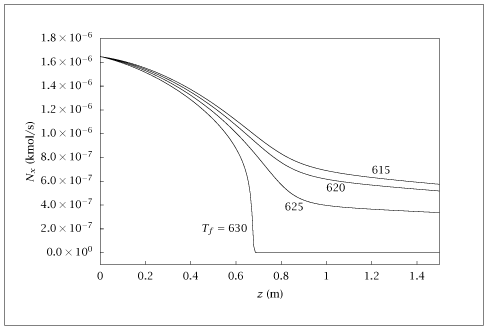## Figure 6.35:

### Molar flow of o-xylene versus reactor length for different feed temperatures.## Code for Figure 6.35

### main.m

```
1
2
3
4
5
6
7
8
9
10
11
12
13
14
15
16
17
18
19
20
21
22
23
24
25
26
27
28
29
30
31
32
33
34
35
36
37
38
39
40
41
42
43
44
45
46
47
48
49
50
51
52
53
54
55
56
57
58
59
60
61
62
63
64
65
66
67
68
69
70
71
72
73
74
75
76
77
78
79
80
81
82
83
84
85
86
87
88
89% Copyright (C) 2001, James B. Rawlings and John G. Ekerdt
%
% This program is free software; you can redistribute it and/or
% modify it under the terms of the GNU General Public License as
% published by the Free Software Foundation; either version 2, or (at
% your option) any later version.
%
% This program is distributed in the hope that it will be useful, but
% WITHOUT ANY WARRANTY; without even the implied warranty of
% MERCHANTABILITY or FITNESS FOR A PARTICULAR PURPOSE.  See the GNU
% General Public License for more details.
%
% You should have received a copy of the GNU General Public License
% along with this program; see the file COPYING.  If not, write to
% the Free Software Foundation, 59 Temple Place - Suite 330, Boston,
% MA 02111-1307, USA.

%
% o-xylene + 3 O_2  -->  phthalic anhydride + 3 H_2O
%
%
% parameters adapted from Welsenaere and Froment, 1970.
%
%
% jbr, 9/21/01
% repaired by jbr, 1/2/10
%
% units: m, kg, kmol, sec
%
% Revised 8/13/2018

p.R     = 1.25e-2; %tube radius, m
p.Ac    = pi*p.R*p.R;  % tube cross-section, m^2
p.Qrho  = 0.0026371; % Ac*4684/3600 mass flow, kg/sec, constant
p.Pf    = 1.01e2;    % feed pressure, kN/m^2, i.e. 1.0 atm
p.Mwf   = 0.98*(0.79*28+0.21*32)+0.02*106.17; % mol wt feed;
% 98%air, 2% o-xylene, kg/kmol
p.Tf    = 625; % feed temperature, K
p.Rg    = 8.314;  % gas constant, kJ/(K kmol)
% p.Nf    = p.Qrho*p.Mwf;  bug here; repaired below; adjust km and delH as well
p.Nf    = p.Qrho/p.Mwf;  % molar flowrate, kmol/sec, also constant
p.E     = 13636.; % activation energy, K
p.Tm    = 625.; % mean temperature,  K
% p.km    = 1922.6;  % 1/sec % adjusted on 1/2/10
p.km    = 2.0822;  % 1/sec
p.Ta    = 625; % coolant temperature, K
p.Cp    = 0.992; % specific heat of mixture, kJ/kg K, assumed constant
% p.delH  = -1.361e3; % kJ/kmol  % adjusted on 1/2/10
p.delH  = -1.284e6; % -3.07e5*4.184 heat of reaction, kJ/kmol, assumed constant
p.U     = 0.373; % heat transfer coefficient, kJ/(m^2 sec K)
p.beta  = p.delH*p.Ac/(p.Qrho*p.Cp);  % heat of reaction parameter,
p.Gamma = 2*pi*p.R*p.U/(p.Qrho*p.Cp); % heat transfer parameter,
p.l     = 1.5; % length of tube, m

npts = 200;
z    = linspace(0, p.l, npts)';
yxfeed = [0.019];
Nxf  = yxfeed*p.Nf;
% Tfeed = [615 625 631 635];
% adjust the temperatures in the repaired file
Tfeed = [615 620 625 630];
nfeed = length(Tfeed);
yx = zeros(npts,nfeed);
T  = zeros(npts,nfeed);

for i = 1: nfeed
Tf = Tfeed(i);
x0 = [Nxf; Tf];

opts = odeset ('AbsTol', sqrt(eps), 'RelTol', sqrt(eps));
[tsolver, x] = ode15s (@(t, x) pfr(t, x, p), z, x0, opts);

yx(:,i) = x(:,1);
T(:,i)  = x(:,2);
end

table = [z yx T];
save -ascii xyleneT.dat table;

if (~ strcmp (getenv ('OMIT_PLOTS'), 'true')) % PLOTTING
subplot(2,1,1);
plot (z, yx);
% TITLE xyleneT

subplot(2,1,2);
plot (z, T);
% TITLE xyleneT_T
end % PLOTTING

```

### pfr.m

```
1
2
3
4
5
6
7
8
9function rhs = pfr(t, x, p)
Nx = x(1);
T  = x(2);
Q  = p.Nf/(p.Pf/(p.Rg*T));
cx = Nx/Q;
k  = p.km*exp(-p.E*(1/T-1/p.Tm));
rate = k*cx;
rhs = [-rate*p.Ac;
-p.beta*rate + p.Gamma*(p.Ta-T)];

```